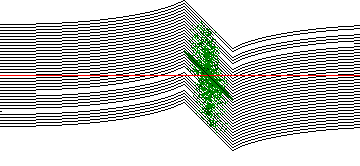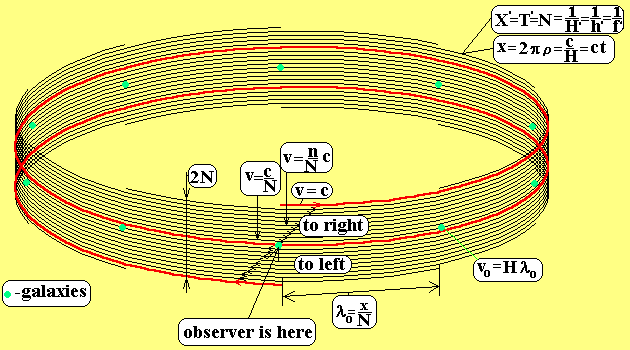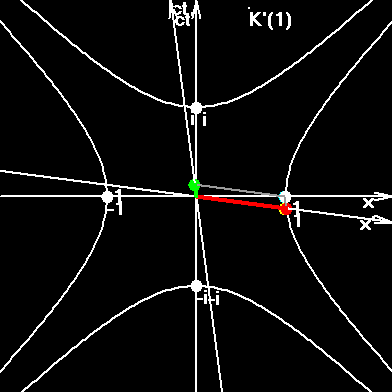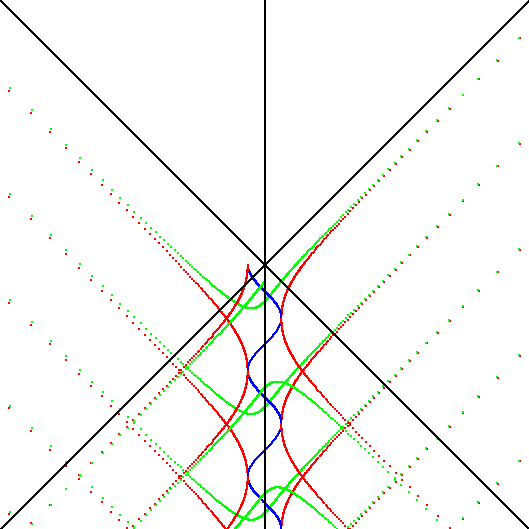# Quantum Gravity and Discrete Space-Time-Matter

Attention! The theory, proposed here, does not coincide to the general adopted views in modern physics.
Gravity is explained here as quantum annihilation of strings, or links of 4d material lattice in the sphere of influence of massive object.

You will see here a very approximate explanation of the quantum gravity "kitchen".

It is supposed in this work that in the smallest vicinity of every space-time point there is a huge quantity of inertial frames of reference (IFR). We suppose here that IFRs are real, or we connect every space line with certain IFR. Consequently, there is no infinity of IFR. If we draw a single line in three-dimensional space, then we must imagine 2N lines situated on this single line. Every two neighboring lines in this bundle of lines move relatively each other with the quantum of velocity v0. The third IFR will move relatively the first IFR with the velocity 2v0. Strictly speaking, we have no rights to add the coordinate velocities, as we had done now. The coordinate velocity has not the property of additivity. But rapidity has such property: vy=vy1+vy2. The analyses had showed that we must quantify another type of velocity, - the quantable velocity, vq. The essence of these four types of velocities is cleared at the pages Some Questions of SR. Here we will make an approximate geometric analyses and we will not pay attention on the type of velocity. Taking into account the upper notes we can say -the top line in the picture has the velocity c=N*v0 to the right, the bottom line has the velocity -c to the left side.In this picture we can see 2N=2*3.09*1020 parallel lines, laying on a single line in the three dimensional space, and which go through of massive object, which is hot inside. We must also imagine two another bundles of lines perpendicular to shown on the picture. Every known stabile elementary particle is a set of two or three defective (absent or extra) links in the lattice. They are moving in circles, embracing such bundles of lines and are materialized as a particle at joining points. The act of materialization we'll name "flash". If flashes occur on one and the same line, IFR, then the particle is in the state of rests in this line. When two successive flashes occur on different lines, then we observe an acceleration, or transition of a particle from one energetic level into another, or transition from one line, IFR, into another line. In the picture the particles are shown as green points. Most of them have small velocities, and we can see them near the central line on the picture. Particles inside of the hot object have larger relative velocities, and we can see them on the top and bottom lines. We supposed that top lines and correspondingly the particles lying (or flashing) on them move to the right; and bottom lines move to the left. The red line corresponds to the state of rest of distant observer. From the left side of the object we can see more lines over the red line, and smaller quantity of lines beneath the red line. That means that from the point of view of a distant observer there are more lines going into object then going out from the object. The phenomenon of gravity is explained here thus: Between two successive flashes, the links rotate on the circumferences, and embrace different quantity of top and bottom lines. Consequently, the next flash will occur near another line. That means the act of acceleration from our point of view; but from the point of view of particle it will be simple not-visible transition from one line of rest into another line of rest.

Every defective link-particle from 4d space, constituting the elementary particle in 3d space, move in such circumference, that inside that circumference there are 2N lines simultaneously. Elementary particle "did not see" the absence of a line from the right or left side. Quite ordinary, that in the end of one revolve the link's trajectories must embrace 2*N lines, and if, suppose, there is no one line from the left or right side, then particle's flash will occur on one level upper or one level bottom relatively the initial line. This new line, IFR, moves to the right or to the left side with the velocity equal to v0=c/N. This means that particle changed its velocity on one minimal step, quantum. It is clear that all particles will have acceleration to the object, as a result of successive transition from one line to another.

Now we'll change the imagination to more complex and more precise form. We must unite circumferences of link-particles and bundles of embraced lines, and as a result, we must come closer to the 4-dimensional (or may be N-dimensional) quasi-closed symmetrical lattice. We draw on the plane 2N lines in such way that they form 2N polygon, but the start point of this polygon and the finish point do not coincide in 4d space on one link along the 4-th coordinate, and do not coincide in 3d space on one time flash. Moreover these lines, forming the local polygon, are also not straight lines, and form the 2N polygons in the closed Universe. In the lattice these two types of polygons are equivalent. We are macroscopic observers, and as a result, from our point of view the mega and micro polygons are different and more the micro-polygons carry different types of particles. But in 4d space the different polygons are equivalent. These polygons we'll name the quasi-closed circumferences. Now we have on the plane 2N lines, every one of which moves with the velocity of light, but if we draw the axis on the plane, then we'll receive the set of projections on the axis with the set of velocities as earlier.

The type of observed elementary particle depends of the angles, which are formed by particle’s circumferences in 4d space to ours coordinate axes. In the times of links joining into proton, neutron, electron, (neutrino), their circumferences are projected into our space not into a continuous lines but into two flashes. (In the space-time the projections are also quasi-circumferences. But in this case the observer must follow behind several constituent parts of a single observed particle simultaneously.) The more distance is between the points of flashes, - the more velocity of observed particles. Photons and gravitons lie on the single scale of fluctuations.

Photon has the frequency more than n0. Graviton has the frequency smaller than n0. This frequency we will name the boundary frequency, and it is equal to 734 Hz. The maximum possible frequency in the lattice corresponds to the stabile particle - proton. The minimum possible frequency corresponds to the Hubble constant, which supposed in this work to be the angular velocity of light. (Particles, which are more massive than proton, are composite or non-stable). Boundary frequency are defined through: n0=sqr(Hnpr). Let's write the frequencies with the help of N:

npr=n0N (1)

H=n0/N (2)

N=sqr(npr/H) (3)

Quasi-closed circumference is shown on the bottom figure.Green points on this picture are galaxies. The red line corresponds to the state of rest of our own Galaxy. Another galaxies are situated in different lines and observers from our Galaxy will see expansion of Universe. But if we remember the absorption (annihilation) of the lines then we'll understand that expansion is constantly compensated by reduction. In this picture we can see as some lines disappear and some lines appear. The average distance between appearances (disappearances) of lines is defined through: l0 = c/n0 = 408 kilometers. At the picture the quantum alteration of velocity (turn in space-time) at the distance l0 is also shown.

v0=Hl0=c/N= 9.7*10-13 m/s (4)

v=Hnl0=cn/N. (5)

### The physical meaning of Hubble constant

It is clear that light makes 4-dimensional rotation. One full rotation needs the time t=1/H. Then velocity of light can be presented as angular velocity measured in radians per second, (rad/s), or as the number of rotations per unit of time (rot/s). It is the Hubble constant.

• c=299792458 m/s

• Number of rotations: H=2.37*10-18 rot/s, (6)

• Angular velocity: 2pH=1.49*10-17 rad/s. (7)

• Turn on one passed meter: 2pH/c=4.98*10-26 rad/m. (8)

• Length of one rotation, or the big circumference of Universe: X=c/H=1.26*1026 m/rot. (10)

In Normalized Units, or in flashes, denoted by !:

• c'=1 !/!

• Number of rotations per !: H' = 1/N rot/!, (11)

• Angular velocity: 2pH' = 2p/N rad/!, (12)

• Rotation on one passed flash: 2pH' = 2p/N rad/!, (13)

• Length of one rotation, or the big circumference of Universe: X'=1/H'=N !. (15)

### Volume of the Universe, and the cosmology constant.

Three-dimensional volume of closed Universe with non identical opposite points can be find with the help of:

V=2p2R3. (16)

(V=p2R3 - with identical opposite points.) Substituting (9) in (16), and then (14) in (16), we'll receive the volume of closed Universe in meters, and in normalized units:

VU = c3/(4pH3), m3. (17)

V'U = c'3/(4pH'3) = N3/4p. (18)

The Universe doesn't expand and don't contract. Massive bodies absorb the space at the sphere of their influence and consume the energy of space. The quantity of swallowed space is equal to the quantity of newborn space. Nevertheless the new space appears mostly in the intergalactic space and disappears mostly near or inside of the massive objects. Let's describe the expansion of intergalactic space.

We have two points A and B at the distance L. The point B moves away from the point A with velocity: v1=HL. After the period of time dt, the point B will move away from point A at the distance: L+dL. Here it will have velocity v2=H(L+dL). The change of velocity will be dv=v2-v1=H(L+dL)-HL=HdL. After dividing the last by dt, we'll receive the acceleration of expansion a=dv/dt=H*dL/dt=H*v1=H*HL=H2L. From the other hand we can write the Newtonian acceleration as: a=4/3*pGrL. The value 4/3*pGr/c2, is named as cosmology coefficient L. If we equal the Newtonian and cosmology accelerations we'll receive another expression for L.

L= H2/c2. (19)

The critical density of matter in the Universe we shall receive, by equating the escape velocity from a surface of some sphere with the radius L, to the velocity of expansion of space. This is correct if we want that the splits of a Big Grenade never condensate together again.

vescape = vHubble

sqr(2GM/L) = HR, M= (4/3)pL3rm

rc = 3H2 / 8pG. (20)

The way to receive the real density is shown on the page Alpha Method, where we had received:

r = rc * W. (21)

r = 3H2 / (8pG) * ((8/3)a)1/2. (22)

r = H2/(pG) * ((3/8)a)1/2. (23)

Knowing the volume of the Universe and its density, we shall receive its mass:

MU= rVU, (24)

### The number N and the forces unification.

N describes:

1. The quantity of IFR in a point;

2. The quantity of ribs in quasi-closed circumference;

3. The quantity of lines embraced by the circumference. In normalized units the following expression is valid:
N=X'=Y'=Z'=T'=1/h'=1/H'=1/f'=1/lP'=1/v0',
where " ' " denotes normalized value;
X, Y, Z, T - dimensions of Universe;
H- Hubble constant, characterizing external rotation, or angular velocity of light;
h- Planck constant, characterizing internal rotation, or quantum of action in electromagnetic region;
f - quantum of force in gravity region;
lpr- Compton wave-length of proton;
v0- quantum of velocity.

4. Expressions of dr M.Geilhaupt unite charge and mass of electron, fine structure constant and his quantum number:
e=sqr(3/2)e0(1-a) - charge,
e0=sqr(e0hc) - fundamental charge,
me=3sqr(2)m0(1-a)/NM - electron mass,
m0=sqr(hc/2pG) - fundamental mass,
a=3/4(1-a)2 - fine structure constant,
e/me=NMsqr(pe0G/6) charge-mass ratio of an electron.

The number NM is connected with N, used in this work, by simple relation NM= sqr(24/a/p)N. Now I'll rewrite Manfred's relations using N, and we'll be able to see how masses of electron and proton are interlaced, how gravity and electromagnetic interactions are interwoven, how microworld and macroworld have united:

Electron mass: mel=sqr(hc/2G)a/N, (25)

The same through H and mpr: mel=hasqr(H/(Gmprc)), (26)

Specific charge of an electron: e/mel=2Nsqr(e0G/a) (27)

N=sqr(apdfe-e), (28)

were dfe-e - electro to gravity forces ratio for two electrons.

The further analysis has shown, that the number N, used here, is proportional to a root square of ND, the hypothetical large number of P.A.M. Dirac. We received it. It is the main quantum characteristic of the lattice of space-time-matter of the Universe.

But the most interesting formulas, uniting different interactions are the following:

aGU = agravity = astrong = aweak = (8/3)ael-magn. (29)

lclass/lBohr=lBohr/lCompton=lCompton/(lRydberg/2)=(8/3)(plScharzschild/lHubble)2=a,

The connection Schwarzschild/Hubble here reminds the connection between the classical radius and the Tomson cross section:
sT =(8/3)prclass2.

1/G' = Exp(a+1/a), (The Earth's Core Is Overheated Emptiness)

To index of Space Genetics,